Readers like you keep news free for everyone.

For the price of one cup of coffee each week you can help keep paywalls away.Dusit via Shutterstock
17 equations that changed the course of humanity
Some you may remember from school, but some are lesser known ones – like one perfected by an Irishman which allowed for development of modern passenger jets.

MATHEMATICIAN IAN STEWART’s recent book In Pursuit of the Unknown: 17 Equations That Changed the Worldtakes a close look at some of the most important equations of all time.

A great example of the human impact of math is the financial crisis. Black Scholes, number 17 on this list, is a derivative pricing equation that played a role.

"It’s actually a fairly simple equation, mathematically speaking," Professor Stewart told us. "What caused trouble was the complexity of the system the mathematics was intended to model."

Numbers have power. In this case, people depended on a theoretical equation too seriously and overreached its assumptions.

Without the equations on this list, we wouldn't have GPS, computers, passenger jets, or countless inventions in between.

You can find the book here.

1. The Pythagorean TheoremWhat does it mean: The square of the hypotenuse of a right triangle is equal to the SUM of the squares of its legs.

History: Attributed to Pythagoras, it isn't certain that he first proved it. The first clear proof came from Euclid, and it is possible the concept was known 1000 years before Pythoragas by the Babylonians.

Importance: The equation is at the core of geometry, links it with algebra, and is the foundation of trigonometry. Without it, accurate surveying, mapmaking, and navigation would be impossible.

Modern use: Triangulation is used to this day to pinpoint relative location for GPS navigation.

2. The logarithm and its identitiesWhat does it mean: You can multiply numbers by adding related numbers.

History: The initial concept was discovered by the Scottish Laird John Napier of Merchiston in an effort to make the multiplication of large numbers, then incredibly tedious and time consuming, easier and faster. It was later refined by Henry Briggs to make reference tables easier to calculate and more useful.

Importance: Logarithms were revolutionary, making calculation faster and more accurate for engineers and astronomers. That's less important with the advent of computers, but they're still an essential to scientists.

Modern use: Logarithms still inform our understanding of radioactive decay.

3. The fundamental theorem of calculus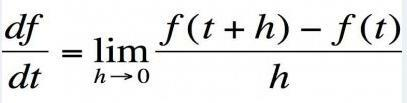What does it mean?: Allows the calculation of an instantaneous rate of change.

History: Calculus as we currently know it was described around the same in the late 17th century by Isaac Newton and Gottfried Leibniz. There was a lengthy debate over plagiarism and priority which may never be resolved. We use the leaps of logic and parts of the notation of both men today.

Importance: According to Stewart, "More than any other mathematical technique, it has created the modern world." Calculus is essential in our understanding of how to measure solids, curves, and areas. It is the foundation of many natural laws, and the source of differential equations.

Modern use: Any mathematical problem where an optimal solution is required. Essential to medicine, economics, and computer science.

4. Newton's universal law of gravitationWhat does it mean?: Calculates the force of gravity between two objects.

History: Isaac Newton derived his laws with help from earlier work by Johannes Kepler. He also used, and possibly plagiarized the work of Robert Hooke.

Importance: Used techniques of calculus to describe how the world works. Even though it was later supplanted by Einstein's theory of relativity, it is still essential for practical description of how objects interact with each other. We use it to this day to design orbits for satellites and probes.

Value: When we launch space missions, the equation is used to find optimal gravitational "tubes" or pathways so they can be as energy efficient as possible. Also makes satellite TV possible.

5. The origin of complex numbersWhat does it mean?: The square of an imaginary number is negative.

History: Imaginary numbers were originally posited by famed gambler/mathematician Girolamo Cardano, then expanded by Rafael Bombelli and John Wallis. They still existed as a peculiar, but essential problem in math until William Hamilton described this definition.

Importance: According to Stewart ".... most modern technology, from electric lighting to digital cameras could not have been invented without them." Imaginary numbers allow for complex analysis, which allows engineers to solve practical problems working in the plane.

Modern use: Used broadly in electrical engineering and complex mathematic theory.

6. Euler's formula for polyhedra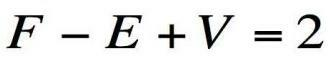What does it mean?: Describes a space's shape or structure regardless of alignment.

History: The relationship was first described by Descartes, then refined, proved, and published by Leonhard Euler in 1750.

Importance: Fundamental  to the development of topography, which extends geometry to any continuous surface. An essential tool for engineers and biologists.

Modern use: Topology is used to understand the behavior and function of DNA.

7. The normal distributionWhat does it mean?: Defines the standard normal distribution, a bell shaped curve in which the probability of observing a point is greatest near the average, and declines rapidly as one moves away.

History: The initial work was by Blaise Pascal, but the distribution came into its own with Bernoulli. The bell curve as we currently comes from Belgian mathematician Adolphe Quetelet.

Importance: The equation is the foundation of modern statistics. Science and social science would not exist in their current form without it.

Modern use: Used to determine whether drugs are sufficiently effective relative to negative side effects in clinical trials.

8. The wave equationWhat does it mean?: A differential equation that describes the behavior of waves, originally the behavior of a vibrating violin string.

History: The mathematicians Daniel Bournoulli and Jean D'Alembert were the first to describe this relationship in the 18th century, albeit in slightly different ways.

Importance: The behavior of waves generalizes to the way sound works, how earthquakes happen, and the behavior of the ocean.

Modern use: Oil companies set off explosives, then read data from the ensuing sound waves to predict geological formations.

9. The Fourier transformWhat does it mean?: Describes patterns in time as a function of frequency.

History: Joseph Fourier discovered the equation, which extended from his famous heat flow equation, and the previously described wave equation.

Importance: The equation allows for complex patterns to be broken up, cleaned up, and analysed. This is essential in many types of signal analysis.

Modern use: Used to compress information for the JPEG image format and discover the structure of molecules.

10. The Navier-Stokes equationsWhat does it mean?: The left side is the acceleration of a small amount of fluid, the right indicates the forces that act upon it.

History: Leonhard Euler made the first attempt at modelling fluid movement, French engineer Claude-Louis Navier and Irish mathematician George Stokes made the leap to the model still used today.

Importance: Once computers became powerful enough to solve this equation, it opened up a complex and very useful field of physics. It is particularly useful in making vehicles more aerodynamic.

Modern use: Among other things, allowed for the development of modern passenger jets.

11. Maxwell's equations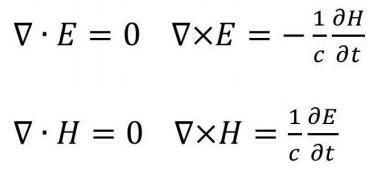What does it mean?: Maps out the relationship between electric and magnetic fields.

History: Michael Faraday did pioneering work on the connection between electricity and magnetism, James Clerk Maxwell translated it into equations, fundamentally altering physics.

Importance: Helped predict and aid the understanding of electromagnetic waves, helping to create many technologies we use today.

Modern use: Radar, television, and modern communications.

12. Second law of thermodynamicsWhat does it mean?: Energy and heat dissipate over time.

History: Sadi Carnot first posited that nature does not have reversible processes. Mathematician Ludwig Boltzmann extended the law, and William Thomson formally stated it.

Importance: Essential to our understanding of energy and the universe via the concept of entropy. It helps us realise the limits on extracting work from heat, and helped lead to a better steam engine.

Modern use: Helped prove that matter is made of atoms, which has been somewhat useful.

13. Einstein's theory of relativity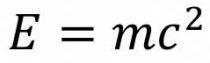What does it mean?: Energy equals mass times the speed of light squared.

History: The less known (among non-physicists) genesis of Einstein's equation was an experiment by Albert Michelson and Edward Morley that proved light did not move in a Newtonian manner in comparison to changing frames of reference. Einstein followed up on this insight with his famous papers on special relativity (1905) and general relativity (1915).

Importance: Probably the most famous equation in history. Completely changed our view of matter and reality.

Modern use: Helped lead to nuclear weapons, and if GPS didn't account for it, your directions would be off thousands of yards.

14. The Schrödinger equationWhat does it mean?: Models matter as a wave, rather than a particle.

History: Louis-Victor de Broglie pinpointed the dual nature of matter in 1924. The equation you see was derived by Erwin Schrodinger in 1927, building off of the work of physicists like Werner Heisenberg.

Importance: Revolutionized the view of physics at small scales. The insight that particles at that level exist at a range of probable states was revolutionary.

Modern use: Essential to the use of the semiconductor and transistor, and thus, most modern computer technology.

15. Shannon's information theoryWhat does it mean?: Estimates the amount of data in a piece of code by the probabilities of its component symbols.

History: Developed by Bell Labs engineer Claude Shannon in the years after World War 2.

Importance: According to Stewart, "It is the equation that ushered in the information age." By stopping engineers from seeking codes that were too efficient, it established the boundaries that made everything from CDs to digital communication possible.

Modern use: Pretty much anything that involves error detection in coding. Anybody use the internet lately?

16. The logistic model for population growthWhat does it mean?: Estimates the change in a population of creatures across generations with limited resources.

History: Robert May was the first to point out that this model of population growth could produce chaos in 1975. Important work by mathematicians Vladimir Arnold and Stephen Smale helped with the realization that chaos is a consequence of differential equations.

Importance: Helped in the development of chaos theory, which has completely changed our understanding of the way that natural systems work.

Modern use: Used to model earthquakes and forecast the weather.

17. The Black-Scholes model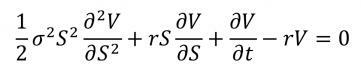What does it mean?: Prices a derivative based on the assumption that it is riskless and that there is no arbitrage opportunity when it is priced correctly.

History: Developed by Fischer Black and Myron Scholes, then expanded by Robert Merton. The latter two won the 1997 Nobel Prize in Economics for the discovery.

Importance: Helped create the now multi trillion dollar derivatives market. It is argued that improper use of the formula (and its descendants) contributed to the financial crisis. In particular, the equation maintains several assumptions that do not hold true in real financial markets.

Modern use: Variants are still used to price most derivatives, even after the financial crisis.

Bonus equations: From an email interview with Dr Stewart.

• At one stage I planned to include the Hodgkin-Huxley equations, which gave mathematical biology a huge boost by using equations to model the way nerve cells send signals to each other. It formed the basis of theoretical neuroscience, and is still important. But it made the book too long, and in the end I felt that its impact on human history has not yet been quite great enough. However, that is likely to change by the middle of this century, as mathematical methods become a major part of mainstream biology — which I think they will.
• My current candidate for and 18th equation (number 1 in ‘Seventeen MORE Equations That Changed the World’ -- I joke... I think...) is the basic equation behind Google. This describes how to rate the importance of a website in terms of the links to it, and it’s a clever application of basic undergraduate linear algebra. It deserved to be in the book, but I was running out of space — and worried that my readers’ enthusiasm for yet another equation might be drying up.

- Max Nisen